## Calculation Sheets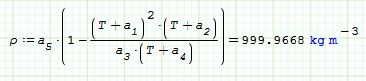This page has been set up to provide hyperlinks to some of my calculation sheets that are stored on the SMath Cloud website. Anyone who wishes to test out the calculation sheets by changing the input values or downloading the files is welcome to do so. If you intend to use the sheets in a professional capacity, I would appreciate an attribution to indicate The Enviro Engineer website. Click on the images to view the calculation sheets.

### Open channel capacity design (Manning's equation)

Hyra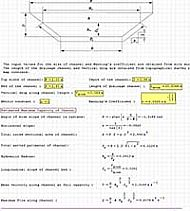ulic computations to determine the mean channel flow velocity and maximum flow capacity in a trapezoidal channel of known size, calculated using the Manning's equations.

### Power & Motion Control Systems

This sh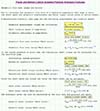eet provides a set of practical hydraulic formulae for estimating hydraulic pump or motor geometric flow rates, shaft torque and hydraulic power. The calculation sheet gives examples of formulae without using a programme such as MathCad or SMath Desktop with units built in and the same equations, but with units included in the calculation.

### Hydraulic Accumulator Sizes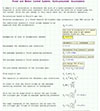This sheet provides a set of sample calculations to determine the size of a hydro-pneumatic accumulator required to store additional hydraulic fluid and avoid the need for a larger pump for the hydraulic system. The model assumes the gas change will be very rapid and there will be adiabatic expansion.

### Open Channel Flow Velocity Empirical Formulae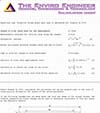Since the earliest time, people have wanted to know how much water could flow through an open channel or closed pipe. Knowing the velocity of a water flow allowed them to determine how large the pipes or channel needed to be to pass the required flow. This might be to allow water to irrigate crops or to supply drinking water to a city.  This sheet shows some of the equations used since the 18th century to estimate the channel flow velocity. This calculation sheet is best used along with my webpage on Open Channel Flow Velocity.

### Mensuration - the theory of measurement of plane figures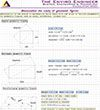This calculation sheet provides a set of practical mathematical equations for estimating the area, perimeter, diagonals and centroid of common geometric plane figures. A description and diagrams of these geometric figures are shown on the Mensuration page of this website.